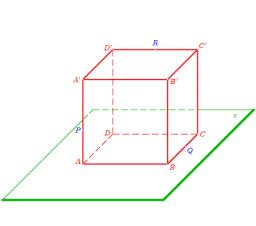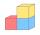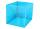# Cube cut

The cube ABCDA'B'C'D ' has an edge of 12cm. Calculate the area of diagonal cut B DD'B '.

S =  203.6468 cm2

### Step-by-step explanation:Did you find an error or inaccuracy? Feel free to write us. Thank you!Tips to related online calculators
Pythagorean theorem is the base for the right triangle calculator.

#### You need to know the following knowledge to solve this word math problem:

We encourage you to watch this tutorial video on this math problem:

## Related math problems and questions:

• Cube diagonalsThe cube has a wall area of 81 cm square. Calculate the length of its edge, wall, and body diagonal.
• Body diagonalFind the cube surface if its body diagonal has a size of 6 cm.
• Body diagonalCalculate the length of the body diagonal of the 6cm cube.
• Cube in sphereThe sphere is an inscribed cube with an edge of 8 cm. Find the sphere's radius.
• Center of the cubeThe Center of the cube has a distance 16 cm from each vertex. Calculate the volume V and surface area S of the cube.
• Body diagonal - cubeCalculate the surface and cube volume with body diagonal 15 cm long.
• CubeCalculate the surface of the cube ABCDA'B'C'D' if the area of rectangle ACC'A' = 344 mm2.
• CalculateCalculate the length of the wall diagonal of the cube with an edge 5 cm long.
• Cuboid easyThe cuboid has the dimensions a = 12 cm, b = 9 cm, c = 36 cm. Calculate the length of the body diagonal of the cuboid.
• Cube diagonalDetermine the length of the cube diagonal with edge 37 mm.
• Body diagonalCalculate the length of the body diagonal of a block with dimensions: a = 20 cm, b = 30 cm, c = 15 cm.
• PrismRight-angled prism, whose base is a right triangle with leg a = 3 cm and hypotenuse c = 13 cm, has the same volume as a cube with an edge length of 3 dm. a) Find the height of the prism b) Calculate the surface of the prism c) What percentage of the cube'
• Cube diagonalsDetermine the volume and surface area of the cube if you know the length of the body diagonal u = 216 cm.I have homework. The cube has an edge 7 cm long and I must find wall and body diagonal.The perimeter of one cube wall is 120 meters. Calculate the surface area and the body diagonal of this cube.Calculate the volume of a regular 6-sided cut pyramid if the bottom edge is 30 cm, the top edge is 12 cm, and the side edge length is 41 cm.The space diagonal of a cube is 129.91 mm. Find the lateral area, surface area and the volume of the cube.